# SAT Math Multiple Choice Question 3: Answer and Explanation

### Test Information

Question: 3

3. If 9−2 =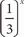x, what is the value of x ?

• A. 1
• B. 2
• C. 4
• D. 6

C Negative exponents mean to take the reciprocal and apply the positive exponent. So 9−2 =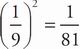. Now find what power of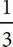equals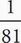. Because 34 = 81,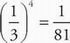, and x must be 4. The correct answer is (C).